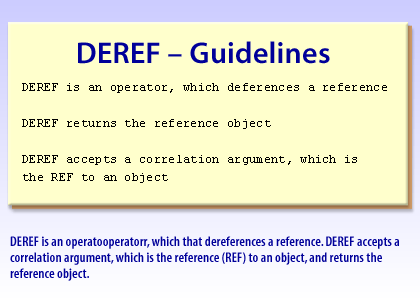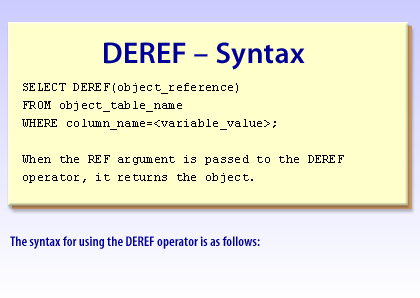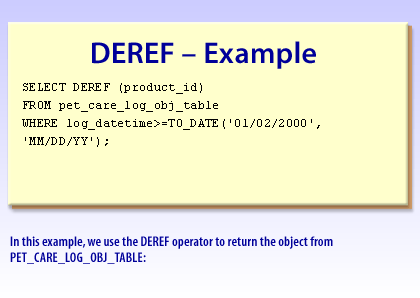PL/SQL   «Prev

# Writing Queries that use DEREF in Oracle

## DEREF syntax

DEREF returns the object instance that the REF value points to. This is more easily explained with a simple example. In this case, DEREF is passed the REF value for the correlation ID of the inventory_tbl:

```SELECT DEREF(REF(i))
FROM inventory_tbl i
WHERE item_id = 1;
```

The object instance associated with the REF value of item_id 1 is returned:
```DEREF(REF(I))(ITEM_ID, NUM_IN_STOCK, REORDER_STATUS, PRICE(DISCOUNT_RA
----------------------------------------------------------------------
INVENTORY_OBJ(1, 10, 'IN STOCK', DISCOUNT_PRICE_OBJ(.1, 75))
```1) DEREF is an operator that dereferences a reference. DEREF accepts a correlation argument, which is the reference (REF) to an object, and returns the reference object. DEREF is an operator that dereferences a reference. DEREF returns the reference object. DEREF accepts a correlation argument, which is the reference (REF) to an object2) Syntax for using the DEREF operator is as follows SELECT DEREF(object_reference) FROM object_table_name WHERE column_name=
When the REF argument is passed to the DEREF operator, it returns the object.3) In this example, we use the DEREF operator to return the object from PET_CARE_LOG_OBJ_TABLE: SELECT DEREF (product_id) FROM pet_care_log_obj_tableWHERE log_datetime>=TO_DATE('01/02/2000', 'MM/DD/YY');